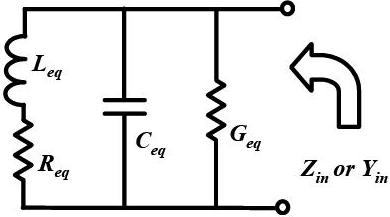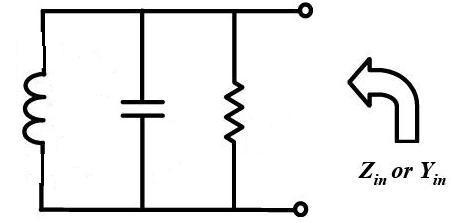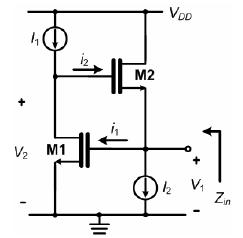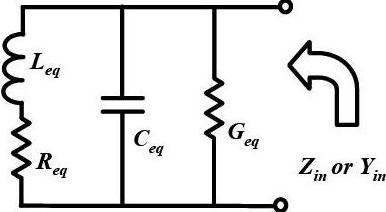# how simulate Q factor in ADS simulator?

Status
Not open for further replies.

#### rezaee

##### Full Member level 2
hello
can anyone help me that how is it possible for me to calculate Q-factor of attached circuit and draw it vs frequency in ADS simulator?Group delay related to loaded Q

QL = (ω0*td)/2

@ E-design : it is just an equation my question is how I simulate in ADS!?

@ pancho_hideboo: firstly I want to use this equation (Q=imag(Zin)/real(Zin)) but I saw it is usable just for RL circuit beacuse Zin=R+LWj and then Q=image(Zin)/real(Zin)=WL/R but we know in a pure parallel RLC circuit Q is equal to W0/2alfa or better I say Q=RCW0 , but if we calculate Q with image(Zin)/real(Zin) causes to different result!!

consider Zin=a+bj then Yin=1/Zin=1/(a+bj) , Yin=[1/(a+bj)]*[(a-bj)/(a-bj)]=(a-bj)/(a^2+b^2) then if you see, just the sign of Image(Zin)/real(Zin) is different from image(Yin)/real(Yin) and the value of them is equal!!
dont you agree with me? its easy you can consider a parallel RLC circuit and calculate Yin(jW0) and then write Q=image(Yin)/real(Yin) and compare it with the Q factor of RLC circuit at W0

ADS can calculate group delay as far as I know. The rest you should be able to implement into an equation and plot.

but how I must do it ? :sad:

OK , but why you delet your last answer posts? this maybe make other reviewers confuse
Later I will repost solution.

#### Attachments

The circuit that I attached in #1 acts as an active inductor now I want to calculate the Q-factor of this, but I dont know how I can do it?
I read if we have an impedance like Zin=a+bj the Q factor is equal to b/a I dont know is it correct or no?
definition of Q-factor for pure parallel RLC is different from the definition of Q-factor at the attached circuit?
for this circuit how I can write formulation of the Q-factor?

The Designer's Guide Community Forum - Bandwidth in Linear PA

The circuit that I attached in #1 acts as an active inductor now I want to calculate the Q-factor of this,
but I dont know how I can do it?
Which do you want to evaluate, Q of inductor or Q of resonator ?

I read if we have an impedance like Zin=a+bj the Q factor is equal to b/a I dont know is it correct or no?
Correct if it is Q of inductor.
The Designer's Guide Community Forum - Inductance extraction: L reduce with frequency?

definition of Q-factor for pure parallel RLC is different from the definition of Q-factor at the attached circuit?
for this circuit how I can write formulation of the Q-factor?
Again which do you want to evaluate, Q of inductor or Q of resonator ?

The Designer's Guide Community Forum - Bandwidth in Linear PA

•rezaee

### rezaee

Points: 2
Surely I want Q of inductor and Q of resonator isnt important for me beacuse its an active inductor,
in this way you mean even the Q that I calculate from image(Zin)/real(Zin) for pure RLC circuit is Q of inductor?

The circuit that I attached in #1 acts as an active inductor now I want to calculate the Q-factor of this, but I dont know how I can do it?

rezaee, I think some information is missing.
Therefore my question: Are all parts values fixed ? (In this case, as pancho-hideboo mentioned, Q is constant).
However, when you intend to tune/vary some elements (e.g. the "active" inductance), the situation has changed a bit.

And - just for clarification: By saying "acts as an active inductor" I suppose you mean, that the Leq-Req combination results from an active realization, right? Can you show us the circuit (opamp or OTA)?

LvW

@ pancho-hideboo: I mean from pure parallel RLC is:@LvW: active inductor circuit schematic is:when put small signal model of transistor I have:I want to calculate equation of Q for this active inductor circuit and also do simulation the Q of it with ADS.

You are still confusing Q of resonator and Q of inductor.

No, I think the whole circuit represents the "active inductor". This simple circuit can do nothing else than to provide a two-pole containing all the parasitics as shown. Thus, in fact it is a resonator rather than an inductor with resistive losses only.
Rezaee, can you confirm?

@pancho_hideboo: my problem is for this circuit(active inductor) that should I use resonator Q or inductor Q!!!?

@LvW: whats your idea? for this circuit(basic gyrator-C active inductor) which Q is important and which of them I should calculate and simulate?

@pancho_hideboo: my problem is for this circuit(active inductor) that should I use resonator Q or inductor Q!!!?

@LvW: whats your idea? for this circuit(basic gyrator-C active inductor) which Q is important and which of them I should calculate and simulate?

Rezaee, I do not understand why you are asking us what to do ("what's your idea"??)
It is YOU, who has to express his desire or goal. How can we know what for you "is important"?
I suppose you want to have an expression for the Q of the whole circuit - that means for the resonator. (But, I only suppose....I do not know!)
Do you expect several (or at least two) alternatives for Q determination? Are you looking for the Q value of an ideal inductor?
I don't think so.
In my reply#17 I have asked you to confirm my assumption. Why don't you respond?

at first I should say beacuse of my english language isnt very good maybe I cant understand some parts of your sentences or maybe understand wrong.
if I ask your idea the reason is that I am newbie in designing and I think you are more skilled.
my final goal is designing an active inductor with high Q, but now I am getting started to do that , firstly I read several thesis,conf and journal papers and patents about this subject and see at first all of them introduced this basic circuit as a conventional active inductor but each of them present different definition of Q , for this reason I got confused beacuse at all of them the circuit is one thing and the goal is one thing (designing active inductor with high Q ) but different definition of Q are used!!

rezaee, if your task is to design a high-Q active inductor I must ask you why you have selected the shown circuit.
Surely, this is not a circuit that can provide the highest possible quality factor.
According to my knowledge the two-opamp circuit (Antoniou-GIC) can realize a high-Q inductor.

Maybe that different authors define different Q factors. However, for my opinion and according to my "feeling" the best and most logical definition would be based simply on real and imaginary part of the one-port (two-pole):
Since the ideal inductor has a pure imaginary impedance Im(Z)=wL (real part Re=0): Q=Im(Z)/Re(Z) with Z=input impedance of the whole two-pole.

Last edited:

You are still confusing Q of resonator and Q of inductor.
No,
Return "No" multiplied by hundred to you.
You can't understand what he is confusing at all.

@ pancho-hideboo: I mean from pure parallel RLC is:
w0*C/G is Q of parallel RLC resonator not Q of inductor.
Here w0=1/sqrt(L*C) is a parallel resonance frequency.

I want to calculate equation of Q for this active inductor circuit and also do simulation the Q of it with ADS.
@pancho_hideboo: my problem is for this circuit(active inductor) that should I use resonator Q or inductor Q!!!?
You have to evaluate Q of inductor.
Strictry speaking, you have to evaluate Q of effective inductor or Q of equivalent series inductive component of DUT.

Idendification of black box as inductor results in what lumped model you apply for a target balck box circuit around frequency you are considering.
The Designer's Guide Community Forum - Inductance extraction: L reduce with frequency?

However in calculation of inductor's Q, we identify black box as simple Zin=Reff + j*w*Leff, Q=w*Leff/Reff=imag(Zin)/real(Zin).
I read if we have an impedance like Zin=a+bj the Q factor is equal to b/a I dont know is it correct or no?
Correct if it is Q of inductor.
The Designer's Guide Community Forum - Negative Impedance seeing Vdd node of Inverters

If you would like to identify parallel RLC resonator as inductor, Q of inductor is calculated by Q=imag(Zin)/real(Zin).
It is never w0*C/G.